# Vector Algebra Class 12 NCERT solutions

## In this pdf file you can see answers of following Questions

### EXERCISE 10.1

Question 1. Represent graphically a displacement of 40 km, 30° east of north.

Question 2. Classify the following measures as scalars and vectors.
(i) 10 kg
(ii) 2 meters north-west
(iii) 40° (iv) 40 watt
(v) 10–19 coulomb
(vi) 20 m/s2

Question 3. Classify the following as scalar and vector quantities.
(i) time period
(ii) distance
(iii) force
(iv) velocity
(v) work done

Question 4. In Fig 10.6 (a square), identify the following vectors.
(i) Coinitial
(ii) Equal
(iii) Collinear but not equal

Question 5. Answer the following as true or false.
(i) a and −a are collin ear.
(ii) Two collinear vectors are always equal in magnitude.
(iii) Two vectors having same magnitude are collinear.
(iv) Two collinear vectors having the same magnitude are equal.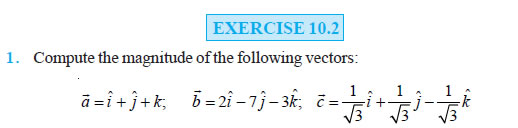Question 2. Write two different vectors having same magnitude.

Question 3. Write two different vectors having same direction.

Question 4. Find the values of x and y so that the vectors 2iˆ + 3 ˆj and xiˆ + yˆj are equal.

Question 5. Find the scalar and vector components of the vector with initial point (2, 1) and terminal point (– 5, 7).

Question 6. Find the sum of the vectors a = iˆ − 2 ˆj + kˆ, b = −2iˆ + 4 ˆj + 5kˆ and c� = iˆ − 6 ˆj – 7kˆ .

Question 7. Find the unit vector in the direction of the vector a = iˆ + ˆj + 2kˆ .

Question 8. Find the unit vector in the direction of vector PQ, where P and Q are the points (1, 2, 3) and (4, 5, 6), respectively.

Question 9. For given vectors, a = 2iˆ − ˆj + 2kˆ and b = −iˆ + ˆj − kˆ , find the unit vector in the direction of the vector a + b .

Question 10. Find a vector in the direction of vector 5iˆ − ˆj + 2kˆ which has magnitude 8 units.

Question 11. Show that the vectors 2iˆ − 3 ˆj + 4kˆ and − 4iˆ + 6 ˆj − 8kˆ are collinear. 12. Find the direction cosines of the vector iˆ + 2 ˆj + 3kˆ .

Question 13. Find the direction cosines of the vector joining the points A(1, 2, –3) and B(–1, –2, 1), directed from A to B .

Question 14. Show that the vector iˆ + ˆj + kˆ is equally inclined to the axes OX, OY and OZ.

Question 15. Find the position vector of a point R which divides the line joining two points P and Q whose position vectors are iˆ + 2 ˆj − kˆ and – iˆ + ˆj + kˆ respectively, in the ratio 2 : 1
(i) internally
(ii) externally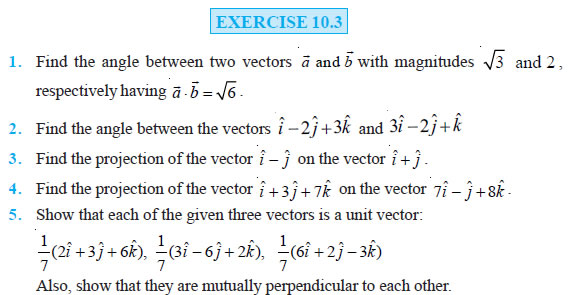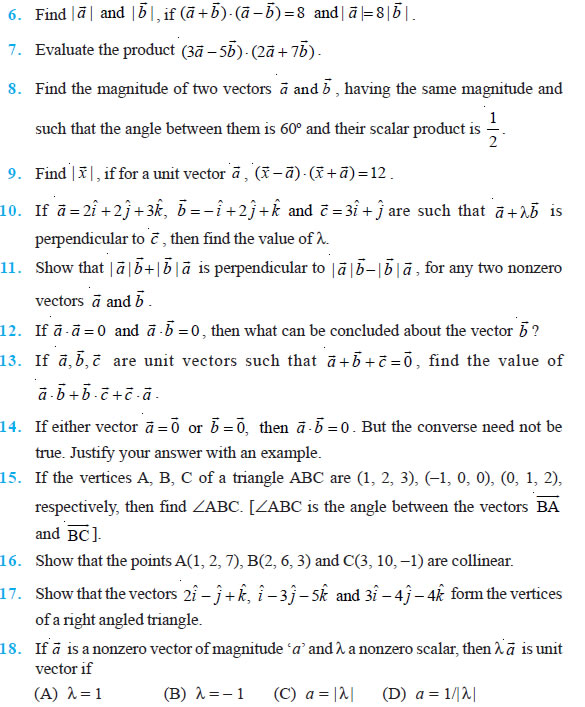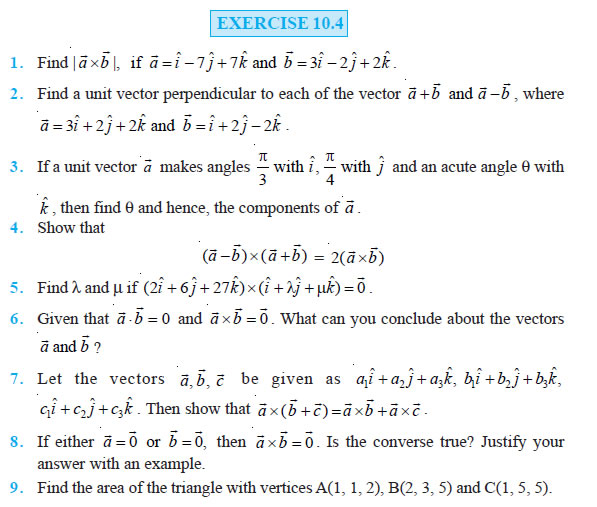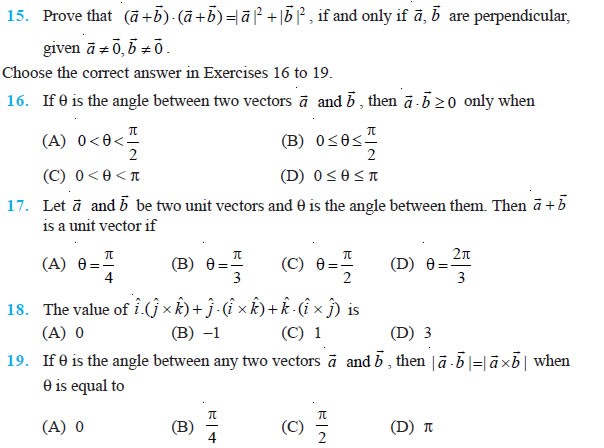### NCERT Books Free Pdf Download for Class 5, 6, 7, 8, 9, 10 , 11, 12 Hindi and English Medium

 Mathematics Biology Psychology Chemistry English Economics Sociology Hindi Business Studies Geography Science Political Science Statistics Physics Accountancy